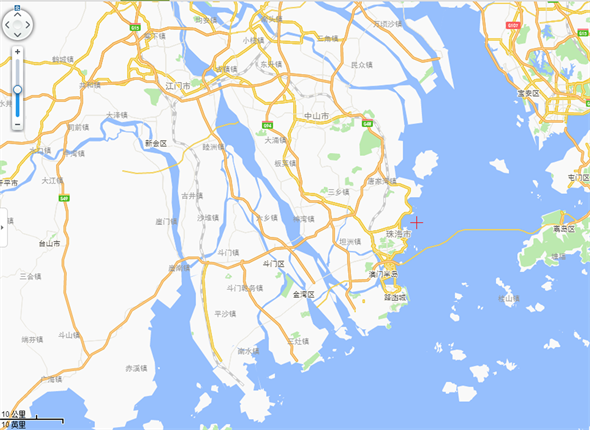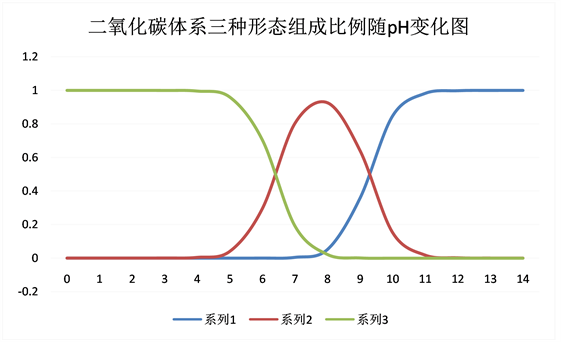# 伶仃洋海水总碱度测定Determination of Total Alkalinity of Lingdingyang Bay

DOI: 10.12677/AMS.2018.52010, PDF, HTML, XML, 下载: 906  浏览: 2,671  国家自然科学基金支持

Abstract: To solve the lack of the alkalinity data of Lingdingyang Bay and Zhuhai coastal area since 1999, the total alkalinity of seawater in the study area was measured by titration method on December 7, 2016. We found that the total alkalinity was 102.10 mg/L (calculated by CaCO3). Compared to the previous data 70.5 mg/L (calculated by CaCO3) , the total alkalinity has been greatly increased. The reasons may be the pollution of domestic sewage, the frequent occurrence of red tide and the increasing domestic sewage pollution.

1. 引言

2. 实验方法

2.1. 样品采集及处理

2.2. 样品总碱度的测定方法Figure 1. Location map of research area

${\text{Na}}_{\text{2}}{\text{CO}}_{\text{3}}+\text{2HCl}=\text{2NaCl}+{\text{CO}}_{\text{2}}+{\text{H}}_{\text{2}}\text{O}$

$\text{NaOH}+\text{HCl}=\text{NaCl}+{\text{H}}_{\text{2}}\text{O}$

${\text{NaHCO}}_{\text{3}}+\text{HCl}=\text{NaCl}+{\text{CO}}_{\text{2}}+{\text{H}}_{\text{2}}\text{O}$

${\text{NaH}}_{\text{2}}{\text{BO}}_{\text{3}}+\text{HCl}=\text{NaCl}+{\text{H}}_{\text{3}}{\text{BO}}_{\text{3}}$

① 量取100.00 ml水样于250 ml锥形瓶中，加入4滴酚酞指示剂，用已经完成标定了的HCl溶液进行滴定至溶液颜色由红色变为无色，当溶液颜色变为无色且半分钟内不发生变化时，酚酞碱度滴定完成，记下HCl溶液的消耗量V1 (ml)；

② 在上述测定过酚酞碱度的水样中，加4滴甲基橙指示剂，用已经完成标定了的HCl溶液滴定至溶液颜色由黄色变为橙色，当溶液颜色变为橙色且半分钟内不发生变化时，甲基橙碱度滴定完成，记下HCl溶液的消耗量V2 (ml)；

③ 计算海水总碱度：以CaCO3 (mg/L)表示水样中酚酞碱度；

3. 结果与讨论

${\delta }_{{\text{HCO}}_{3}^{-}}=\frac{\left[{\text{H}}^{+}\right]{K}_{a1}}{{\left[{\text{H}}^{+}\right]}^{2}+\left[{\text{H}}^{+}\right]{K}_{a1}+{K}_{a1}{K}_{a2}}=97.2%$

${\delta }_{{\text{H}}_{\text{2}}{\text{CO}}_{\text{3}}}=\frac{{\left[{\text{H}}^{+}\right]}^{2}}{{\left[{\text{H}}^{+}\right]}^{2}+\left[{\text{H}}^{+}\right]{K}_{a1}+{K}_{a1}{K}_{a2}}=2.3%$

${\delta }_{{\text{CO}}_{3}^{2-}}=\frac{{K}_{a1}{K}_{a2}}{{\left[{\text{H}}^{+}\right]}^{2}+\left[{\text{H}}^{+}\right]{K}_{a1}+{K}_{a1}{K}_{a2}}=0.5%$Figure 2. The change of the proportion of three forms of carbon dioxide with pH)Table 1. Results of total alkalinity measurement of sea water in 2016

2000年之后，城市居民大幅增加，而污水处理设施发展滞后，富含有机物和营养盐的居民生活污水成为珠江口海域的主要污染源，占总污水量的70%，导致珠江口大面积海域水质处于Ⅳ级水质  ，水域富营养化严重。对于我们的测量位点，营养盐测量值DIN = 127.2 μmol/l， ${\text{PO}}_{4}^{3-}\text{-P}$ = 1.00 μmol/l，处于Ⅵ级营养级(磷限制潜在性富营养)(吴斌斌等2010)  。营养盐污染严重的同时有机物的污染十分严重，澳门一侧沉积物中毒害有机物含量为珠江口最高  。

1) 从盐度影响来看。采样时珠海正处于低潮期，河水冲淡的作用相对较强，近岸海水盐度处于较低值，因海水盐度而导致高碱度的可能较低。

2) 从CaCO3的沉淀和溶解来看。从碳酸盐的沉淀与溶解来看。采样点海域曾属于贝类养殖区域  ，有大量的人为甲壳废弃物，可能对附近水域碱度产生影响。

3) 氮的生物吸收和再矿化过程中溶解无机氮的释放。

4) 河流水量变化和降水情况。根据表2所示，采样前一周珠海市均没有发生过降水，不存在外来降水的冲淡，而且珠江处于枯水期，水量较小。海水对近岸水体的影响较大，可能使碱度升

4. 结论

2016年12月7日伶仃洋珠海沿岸海水总碱度测量值为102 mg/L (以CaCO3计)，对比发现海水总碱Table 2. Weather data of Zhuhai City (from The Weather of China)Table 3. Results of total alkalinity measurement of sea water in 2015Table 4. Weather data of Zhuhai City (from The Weather of China)

  李福荣, 陈国华, 纪红. 珠江口海水碱度研究[J]. 青岛海洋大学学报(自然科学版), 1999(S1): 49-54.  臧维玲. 海水的缓冲性能及缓冲容量[J]. 海洋科学, 1983(4): 18.  陏永年. 海水总碱度及其示性特征[J]. 山东海洋学院学报, 1986(1): 160-172+208.  胡祎, 肖瑜, 彭雪妍, 张敏, 章波, 李中秋. 海洋酸化研究进展[J]. 水科学与工程技术, 2011(1): 19-21.  王爱军, 王聪, 石超英. Gran滴定法测量不同盐度海水样品的总碱度浓度及其影响因素[J]. 海洋技术学报, 2014(2): 81-86.  赵小芳, 侯纯扬, 樊利华, 崔振东. 海水及浓海水吸收二氧化硫[J]. 环境工程学报, 2013, 7(8): 3123-3130.  梁志, 王肇鼎. 珠江口氯度、盐度、电导和碱度的状况与相互关系[J]. 海洋学报(中文版), 1983(6): 728-734.  彭云辉, 陈浩如, 李少芬. 珠江河口水体的pH和碱度[J]. 热带海洋, 1991(4): 49-55.  窦勇, 高金伟, 时晓婷, 陈瑞楠, 周文礼. 2000~2013年中国南部近海赤潮发生规律及影响因素研究[J]. 水生态学杂志, 2015(3): 31-37.  韦桂秋, 王华, 蔡伟叙, 易斌. 近10年珠江口海域赤潮发生特征及原因初探[J]. 海洋通报, 2012(4): 466-474.  贺青. 厦门内湾赤潮多发区的AOI和pH用于赤潮预警的探讨[D]: [硕士学位论文]. 厦门: 国家海洋局第三海洋研究所, 2010.  魏晓霞. 庆阳市地下水中碱度的测定[J]. 宝鸡文理学院学报(自然科学版), 2016(4): 59-61, 78.  彭云辉, 王肇鼎, 易志伟. 珠江河口水体的二氧化碳[J]. 环境化学, 1992(4): 56-60.  应秩甫, 陈世光. 珠江口伶仃洋咸淡水混合特征[J]. 海洋学报(中文版), 1983(1): 1-10.  韩舞鹰, 等. 珠江口海水的碳贮量[J]. 环境科学, 1990, 11(2): 12-17.  贾后磊, 谢健, 吴桑云, 何桂芳. 近年来珠江口盐度时空变化特征[J]. 海洋湖沼通报, 2011(2): 142-146.  何颖清, 喻丰华, 杨留柱, 尹斌. 珠江口水表盐度遥感反演及空间分异特点分析[J]. 人民珠江, 2012(S1): 52-55.  何桂芳, 袁国明, 李凤岐. 珠江口沿岸城市经济发展对珠江口水质的影响[J]. 海洋环境科学, 2004(4): 50-52+70.  吴斌斌, 高全贺, 李衍森. 珠海近岸水体营养盐污染状况及潜在性富营养化程度评价[J]. 科技资讯, 2010(4): 134-135.  刘芳文, 颜文. 珠江口及其邻近水域的化学污染研究进展[J]. 海洋科学, 2002(6): 27-30.  陈敏. 影响总碱度的海洋学过程[J]. 化学海洋学, 2009: 75.  张亚锋, 雷俊, 郑晓坤, 黄志坚. 珠海牡蛎养殖业现状初步调查分析和思考[J]. 水产科技, 2010(Z1): 21-23.  邓孺孺, 何执兼, 陈晓翔, 关履基, 柯栋. 珠江口水域水污染遥感定量分析[J]. 中山大学学报(自然科学版), 2002(3): 99-103.  尹姗姗, 朱雅敏. 珠海市近岸海域水质综合评价及防污措施研究[J]. 水利科技与经济, 2014(2): 26-29.  李骞. 春夏季东海赤潮高发区颗粒有机物的来源、含量变化及其与固碳作用的关系[D]: [硕士学位论文]. 青岛: 中国海洋大学, 2007.  陈翩翩. 去年珠海水域发生两次赤潮[N]. 珠海特区报, 2016-06-14(008).  宋雪梅. 实行最严格环境保护制度[N]. 珠海特区报, 2017-01-24(003).